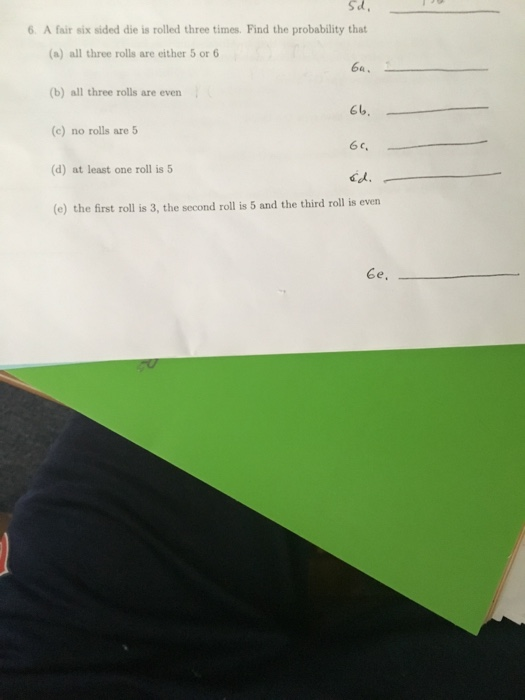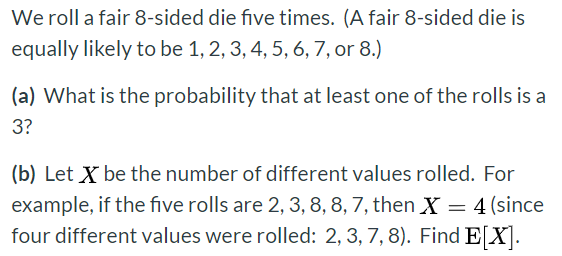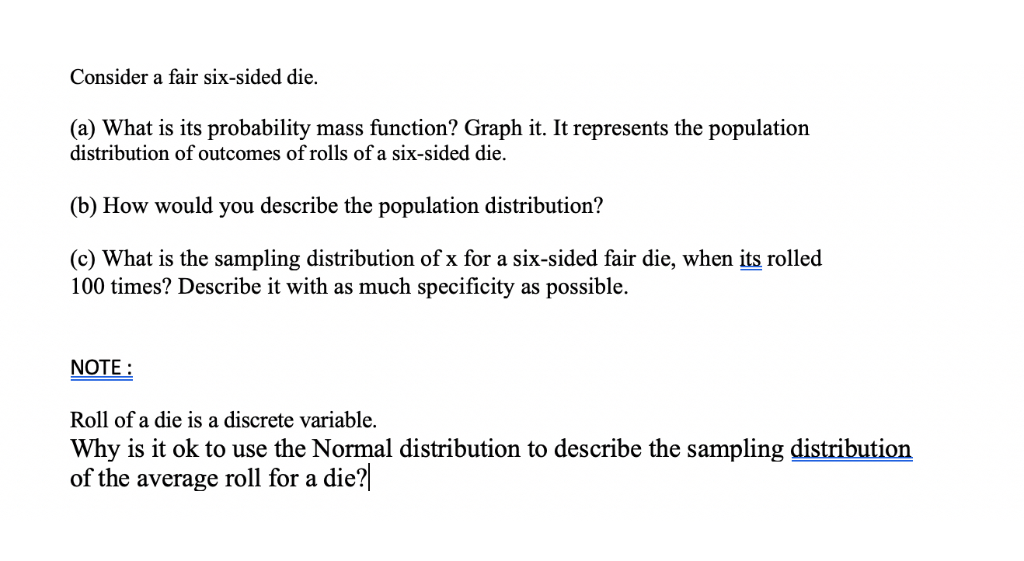Questiona)as 5 ,6 are two outcomes out of 6 , therefore P(a single die shows 5 or 6) =2/6

P(all three are either 5 or 6) =(2/6)*(2/6)*(2/6)=1/27
b P(all three are even) =(1/2)*(1/2)*(1/2)=1/8

c) P(no rolls are 5) =(5/6)*(5/6)*(5/6) =125/216

d) P(At least one is 5) =1-P(none is 5) =1-125/216 =91/216

e) Probability= (!/6)*(1/6)*(3/6) =1/72

#### Earn Coins

Coins can be redeemed for fabulous gifts.

Similar Homework Help Questions
• ### A six-sided die is to be rolled three times. Assume the rolls are independent and that...

A six-sided die is to be rolled three times. Assume the rolls are independent and that the die is fair. - The probability that all three rolls result in an even number is: A) 1.0 B) 0.75 C) 0.25 D) 0.125 - The probability that at least one of the rolls is an even number is: A) 0.125 B) 0.333 C) 0.750 D) 0.875 - The events A = exactly two of the rolls are even and B = exactly...

• ### A fair 6-sided die rolled 5 times. what is the probability that at least one of...

A fair 6-sided die rolled 5 times. what is the probability that at least one of the rolls is 2

• ### Suppose I asked you to roll a fair six-sided die 6 times. You have already rolled...

Suppose I asked you to roll a fair six-sided die 6 times. You have already rolled the die for 5 times and six has not appeared ones. Assuming die rolls are independent, what is the probability that you would get a six in the next roll? 1/6 1/2 5/6 0 1

• ### We roll a fair 8-sided die five times. (A fair 8-sided die is equally likely to...We roll a fair 8-sided die five times. (A fair 8-sided die is equally likely to be 1, 2, 3, 4, 5, 6, 7, or 8.) (a) What is the probability that at least one of the rolls is a 3? (b) Let X be the number of different values rolled. For example, if the five rolls are 2, 3, 8, 8, 7, then X = 4 (since four different values were rolled: 2,3,7,8). Find E[X].

• ### If a fair six sided die is rolled three times....

1) If a fair six-sided die is rolled three times and the number of spots on the resulting upward face is observed for each roll, what is the probability that the 2ndnumber observed will be greater than the 1st number observed and the 3rd number observed will be equal to the sum of the first two numbers observed? (Make the usualassumptions concerning fair dice.)(Hint: Noting that we can work with a sample space having equally-likely outcomes, the probability can be...

• ### A six sided die is rolled three times

A six sided die is rolled three times. List possible outcomes and find the probability of rolling exactly one 6.

• ### Three six-sided fair dice are rolled. The six sides are numbered 1,2,3,4,5,6. Let A be the...

Three six-sided fair dice are rolled. The six sides are numbered 1,2,3,4,5,6. Let A be the event that the first die shows an even number, let B be the event that the second die shows an even number, and let C be the event that the third die shows an even number. Express each of the following events in terms of the named events described above: 1) the event that all three dice show even numbers 2) the event that...

• ### What is the probability that a fair six-sided die rolled five times comes up 6 exactly...

What is the probability that a fair six-sided die rolled five times comes up 6 exactly once?

• ### Three fair six-sided dice are rolled. a) What is the probability of seeing {1, 3, 6}?...

Three fair six-sided dice are rolled. a) What is the probability of seeing {1, 3, 6}? b) What is the probability of seeing {1, 4, 4}? c) What is the probability of seeing {2, 2, 2} ? d) What is the probability of seeing at least one 6? e) What is the probability that the sum of all three dice is 16? f) What is the probability of seeing exactly two even numbers?

• ### Consider a fair six-sided die. (a) What is its probability mass function? Graph it. It represents...Consider a fair six-sided die. (a) What is its probability mass function? Graph it. It represents the population distribution of outcomes of rolls of a six-sided die (b) How would you describe the population distribution? (c) What is the sampling distribution of x for a six-sided fair die, when its rolled 100 times? Describe it with as much specificity as possible. NOTE: Roll of a die is a discrete variable. Why is it ok to use the Normal distribution to...